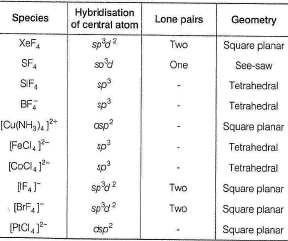# Test: Chemical Bonding (VSEPR Model) And Hybridisation

## 26 Questions MCQ Test Chemistry Class 11 | Test: Chemical Bonding (VSEPR Model) And Hybridisation

Description
This mock test of Test: Chemical Bonding (VSEPR Model) And Hybridisation for Class 11 helps you for every Class 11 entrance exam. This contains 26 Multiple Choice Questions for Class 11 Test: Chemical Bonding (VSEPR Model) And Hybridisation (mcq) to study with solutions a complete question bank. The solved questions answers in this Test: Chemical Bonding (VSEPR Model) And Hybridisation quiz give you a good mix of easy questions and tough questions. Class 11 students definitely take this Test: Chemical Bonding (VSEPR Model) And Hybridisation exercise for a better result in the exam. You can find other Test: Chemical Bonding (VSEPR Model) And Hybridisation extra questions, long questions & short questions for Class 11 on EduRev as well by searching above.
QUESTION: 1

### Direction (Q. Nos. 1-16) This section contains 16 multiple choice questions. Each question has four choices (a), (b), (c) and (d), out of which ONLY ONE option is correct. Q.  The shape [CIF4]- and [ClF2]- ions is respectively

Solution: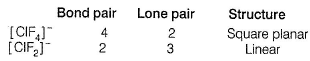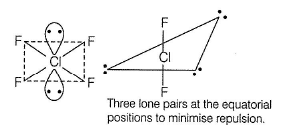QUESTION: 2

### Which of the following sequences shows the correct bond angle order for isoelectronic species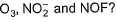Solution: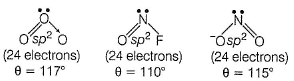In each central atom is sp2-hybridised, each having one lone pair.
Difference in the extent of repulsion changes value of  θ.

QUESTION: 3

### XeF2 is isostructural with

Solution:

XeF2-sp3d hybridised Xe
Three lone pairs on equatorial positions to minimise repulsion; two F-atom at axial position. Thus, it is linear.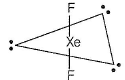(a) TeF2,Te50 - Six electrons in valence shell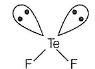(b)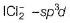hybridised l- atom with three lone pairs at equatorial positions to minimise repulsion thus, linear.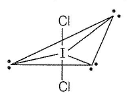(c) SoCI3 - sp3-hybridised pyramidal Sb-one lone pair on Sb.
(d) BaCI2 - ionic .

QUESTION: 4

The species having pyramidal shape is

[IIT JEE 2010]

Solution: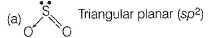(b) BrF3 - T-shaped (sp3d2)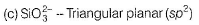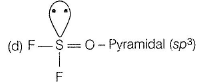QUESTION: 5

Molecular shape of CF4, SF4 and XeF4 are

Solution: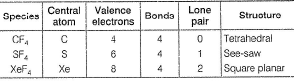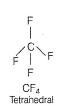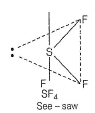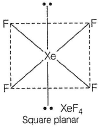QUESTION: 6

Two of the following species have same shape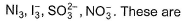Solution: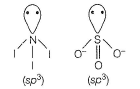Both have one lone pair-trigonal pyramidal.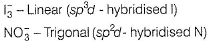QUESTION: 7

ECI3 (where, E = B, P, As, Bi) of these elements are known.
Bond angles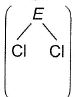are in the following order

Solution: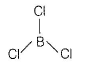Has no lone pair thus, bond angle is 120°. B-atom is sp2-hybridised.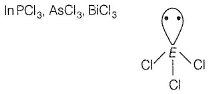Central atom E is sp3-hybridised. Hence, angle < 120°. As we go down the group, (Ip-bp) repulsion decreases. Hence, angle
(Cl—E—Cl) PCI3 > AsCI3 > BiCI3.
Thus, order is BCI3 > PCI3 > AsCI3 > BiCI3.

QUESTION: 8

Consider the two structures :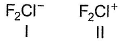Select the correct statement(s).

Solution:

1 has three lone pair and two bond pair, its representation will be AB2L3 and according to VSEPR theory it is linear in shape.

2 has two LP and two BP which representation will be AB2L2, so according to VSEPR theory it will be bent or it can also said to be 'V' shaped.

Hence B is correct.

QUESTION: 9

Which of the two ions from the list given below that have the geometry that is explained by the same hybridisation of orbitals?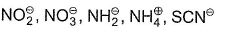Solution: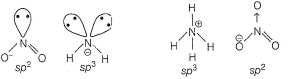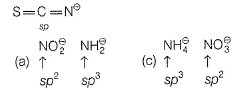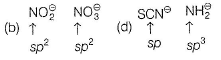QUESTION: 10

The correct order of increasing bond angles in the following species is

Solution: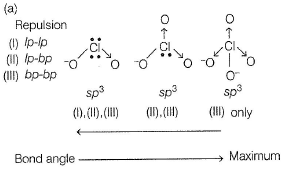QUESTION: 11

Which of the following molecule/species has the minimum number of lone pairs?

Solution: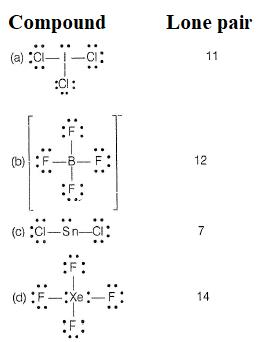QUESTION: 12

PCI5 has a shape of trigonal bipyramid whereas, IF5 has a shape of square pyramid. It is due to

Solution:

PCI5
sp3d-hybrid orbitals—No non-bonding pair trigonal bipyramidal structure.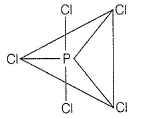IF5 sp3d2-hybrid orbitals—One non-bonding pair; one F and non-bonding pair lie in different sides so as to minimise repulsion. Square pyramidal.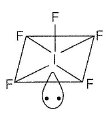QUESTION: 13

For H2O and H2S, given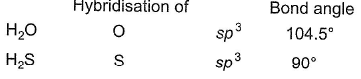Q.

Difference in bond angle is due to

Solution: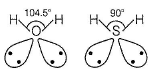There is (i) Ip - Ip (ii) Ip - bp (iii) bp - bp repulsion
Thus, bond angle is less than 109.5°. In H2O, 104.5° > 90° in H2S. It is due to larger size of S-atom which minimises the repulsion and allows the bonds in H2S to be purely p-type.

QUESTION: 14

For which of the following sets of geometry, both axial and equatorial positions are present?

Solution: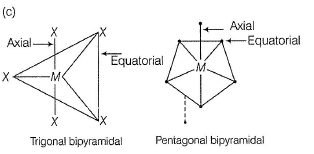QUESTION: 15

Which of the following set of molecules have the same shape but different hybridisation?

Solution: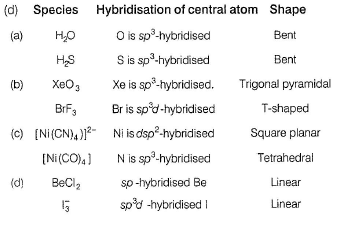QUESTION: 16

Which is the correct order of the bond angle?

Solution: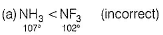The high electronegativity of F pulls the bonding electrons farther away from N than in NH3. Thus, repulsion between bond pairs is iess in NF3 than in NH3. Flence, the lone pair in N causes a greater distortion than NH3.
(b) as in (a)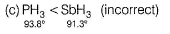Going down the group in periodic table, as size of central atom increases, repulsion increases.

*Multiple options can be correct
QUESTION: 17

Direction (Q. Nos. 17-20) This section contains 4 multiple choice questions. Each question has four choices (a), (b), (c) and (d), out of which ONE or  MORE THANT ONE  is correct.

Q.

Which of the following statements are correct regarding Cl2O molecule?

Solution: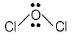O-atorn is sp3-hybridised and thus, bent due to presence of lone pairs on O-atom molecule is polar and planar.

*Multiple options can be correct
QUESTION: 18

In AX4E2, atom A is surrounded by four atoms X and two lone pairs on it. This type of structure is predicted in

Solution: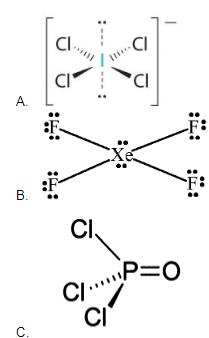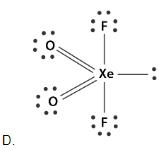Hence A and B only

*Multiple options can be correct
QUESTION: 19

Which of the following sets of molecules have different shape but same hybridisation of the central atoms?

Solution:

NH3   N atom sp3 -hybridised   - Bipyramidal
H2O   O a to m sp3 -hybridised   - V -shaped
H2S   S atom is sp3 -hybridised   - Bent
SO2   S atom is sp2 -hybridised   - Bent
Ni(CO)4   Ni atom is sp3-hybridised   - Tetrahedral
[NiCI4]2-  Ni atom is sp3-hybridised   -Tetrahedral.
SF4   S atom is sp3d -hybridised   - See-saw
PCI5   P atom is sp3 -hybridised   - Bipyramidal

*Multiple options can be correct
QUESTION: 20

Select the correct statement(s) about IF7.

Solution: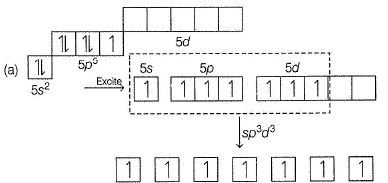Hybridisation of l-True
(b) Oxidation state of I = + 7 (highest) -True.
(c) It has two types of bonds two axial (shortest) and five equatorial (longest) - True.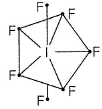(d) Pentagonal bipyramidai structure - True.

QUESTION: 21

Direction (Q. Nos. 21-22) This section contains  a paragraph, wach describing theory, experiments, data etc. three Questions related to paragraph have been given.Each question have only one correct answer among the four given options (a),(b),(c),(d).

Following reaction, CIF3 + AsF5 → (CIF2+ ) (AsF-6)

Q.

Select the correct statements.

Solution: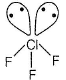sp3d -hybridised Cl-atom - (T-shaped s tructure)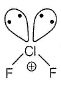sp3 -hybridised. Cl-atom (Bent)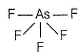sp3d -hybridised As-atom (Trigonal bipyramidai).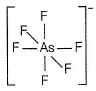sp3d2 -hybridised As-atom (Octahedral).

QUESTION: 22

Following reaction, CIF3 + AsF5 → (CIF2+ ) (AsF6-)

Q.

Select the correct statement(s).

Solution:sp3d -hybridised Cl-atom - (T-shaped s tructure)sp3 -hybridised. Cl-atom (Bent)sp3d -hybridised As-atom (Trigonal bipyramidai).sp3d2 -hybridised As-atom (Octahedral).

QUESTION: 23

Direction (Q. Nos. 23 and 24) Choice the correct combination of elements and column I and coloumn II  are given as option (a), (b), (c) and (d), out of which ONE option is correct.

Q.

Match the species in Column I with the structure in Column II.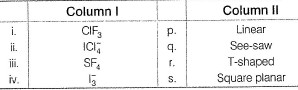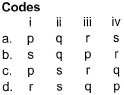Solution: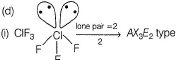Based on VSEPR model, it is (T-shaped).
Thus, (i) → (r)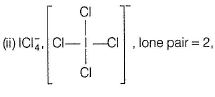AX4E2 (Square planar)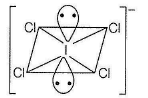Thus, (ii) → (s)
(iii)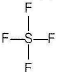lone pair = 1, AX4E (See-saw)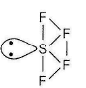Thus, (iii) → (q)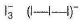lone pair = 3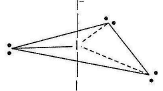Linear structure
Three lone pairs are at equatorial position to minimise repulsion.
Linear structure.
Three lone pairs are at equatorial position to minimise repulsion.
Thus, (iv) → (p)

QUESTION: 24

Match the species in Column I with the shape in Column II.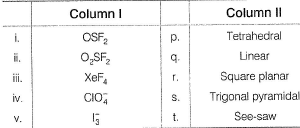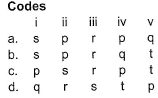Solution:

(i) OSF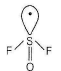sp3-hybridised S -atom (Ip - bp) repulsion, trigonal pyramidal
(ii)→ (s)
(ii) O2SF2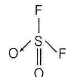sp3-hybridised s-atom tetrahedral
(ii) → (P)
(iii) XeF4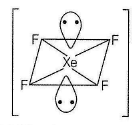sp3d2-hybridised Xe-atom.
Two lone pairs, square planar (iii) → (r)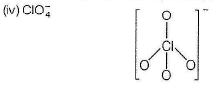sp3-hybridised Cl atom, tetrahedral.
(iv) → (p)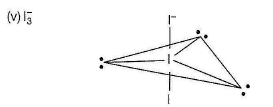sp3d-hybridised I, three lone pairs at equatorial position linear,
(v) → (q)

*Answer can only contain numeric values
QUESTION: 25

Direction (Q. Nos. 25 and 26) This section contains 2 questions. when worked out will result in an integer from 0 to 9 (both inclusive).

Q.

i. Based on VSEPR theory, the number of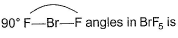ii. Based on VSEPR theory, number of lone pairs in XeF2 is......
iii. The total number of lone pair of electrons in melamine i s ......

Solution:

(i) (0)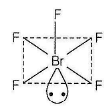(Ip-bp) repulsion will cause distortion and thus, bond angle < 90° in each.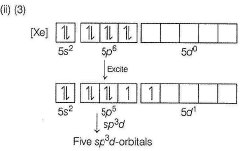Thus, three lone pairs at equatorial position to minimise repulsion.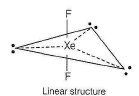(iii) (6) Melamine is heterocyclic basic com pound (six N-atoms) each N having one lone pair.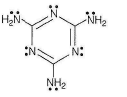*Answer can only contain numeric values
QUESTION: 26

A list of species having the formula XZ4 is given below :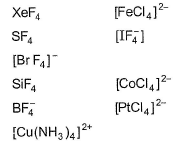Defining the shape on the basis of location X and Z, the total number of species having a square planar shape is .......